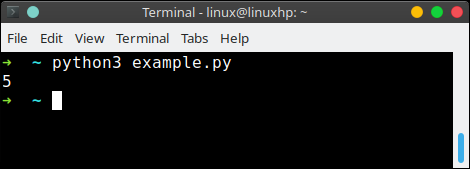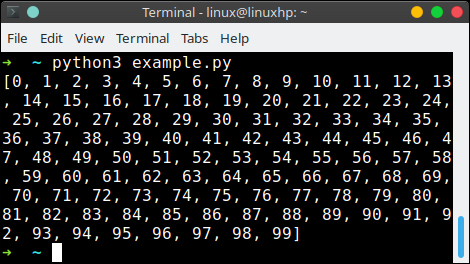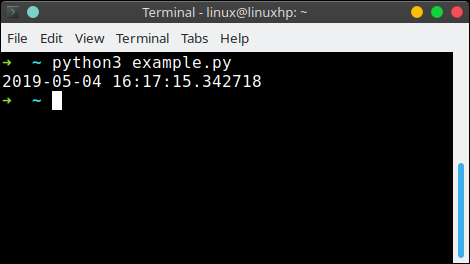tag: beginner | Python Tutorial

# Tag: beginner

## Lists

A list is a collection of objects

Everything in Python is an object. These objects in a list are numbers in most cases. Lists can also contain text (strings) or both.

Note: Lists can have zero or more elements (empty lists are possible).

Related Course:

## Lists Example

A list is defined using square brackets. Let us define as simple list:

In this case we have a list defined by the variable c. We defined a purely random set of numbers above.

## Accessing elements

To access individual elements, we use the same brackets. To print the first element (Python starts counting from zero):

This showsTo print the second element:

Results in:

2

To print the last element, you can count from the back using the minus sign.

Result

18

## List size

You can get the length of the list using the len function. Example code:

## Datatypes

List may contain various types of variables in the same list including text, whole numbers, floating point numbers and so on. For example, we may define a list with text variables:

## List comprehensions

What is list comprehension?

List comprehensions are an easy way to create lists.

Its much easier to write a one liner than it is to write a for loop, just for creating a list. This _ one liner_ is called a list comprehension.

Related Course:

## list comprehension example

Assume we want to create a list containing 100 numbers. Manually that would be a lot of typing work. So we would use a for loop, right?

We can define a for loop to fill the list.

We can replace it with a one liners, which is how we obtain the same result:This is also useful if you want to create large lists.

Note: List comprehensions can include function calls and expressions.

Assume we want the square roots to 100:

## If statements

If statements are all about choices.

A block of code is executed based on one or more conditions. The block of code will only be executed if the condition is true.

Related Course:

## If statements

Computer programs do not only execute instructions. Occasionally, a choice needs to be made. Such as a choice is based on a condition.

Python has several conditional operators:

Conditions are always combined with variables. A program can make a choice using the if keyword. For example:

The condition is shown on line 3.

When you execute this program it will always print ‘End of program’, but the text ‘You guessed correctly!’ will only be printed if the variable x equals to four (see table above).

Note: Code is executed based on the variable x. Try different numbers: 2,3,4

## If else

Python can also execute a block of code if x does not equal to 4. The else keyword is used for that.

If x is set to 2, the second code block is executed. If x equals (==) four, the first code block is executed.

Take a look at the execution below:## datetime

To print the current date, we can utilize the datetime module. Dates are objects, like anything in Python. Datetime has several subclasses, which can be confusing at first.

Related course:

## Date Example

We can use its method `strftime()` to change the format.
To show the current date (today):

This will show the date in the format

YYYY-MM-DD

If you want another format, use the method strftime():
This will output

DD-MM-YYYY

## Get current time in Python

You can get the current time like this:

To get the date and time, use:## Format codes

When using the method strftime() we can specify the type of date format we want to show.

So which format codes do we have?

Format CodeMeanining
%aLocale’s abbreviated weekday name.
%ALocale’s full weekday name.
%bLocale’s abbreviated month name.
%BLocale’s full month name.
%cLocale’s appropriate date and time representation.
%dDay of the month as a decimal number [01,31].
%fMicrosecond as a decimal number [0,999999], zero-padded on the left
%HHour (24-hour clock) as a decimal number [00,23].
%IHour (12-hour clock) as a decimal number [01,12].
%jDay of the year as a decimal number [001,366].
%mMonth as a decimal number [01,12].
%MMinute as a decimal number [00,59].
%pLocale’s equivalent of either AM or PM.
%SSecond as a decimal number [00,61].
%UWeek number of the year (Sunday as the first day of the week)
%wWeekday as a decimal number [0(Sunday),6].
%WWeek number of the year (Monday as the first day of the week)
%xLocale’s appropriate date representation.
%XLocale’s appropriate time representation.
%yYear without century as a decimal number [00,99].
%YYear with century as a decimal number.
%zUTC offset in the form +HHMM or -HHMM.
%ZTime zone name (empty string if the object is naive).
%%A literal ‘%’ character.

## Random Numbers

The random module can be used to make random numbers in Python. The function random() generates a number between 0 and 1.

Online Courses

## Generate random nubers

Generate a real number between 0 and 1
Simply call the random() method to generate a real (float) number between 0 and 1.

Generate a number between 0 and 50
We use the randint() method to generate a whole number.

Generate a random number between 1 and 10
Change the parameters of randint() to generate a number between 1 and 10.

## List of random numbers

To generate a list of 100 random numbers:

## Choosing random items from a list

To get 3 random items from a list: